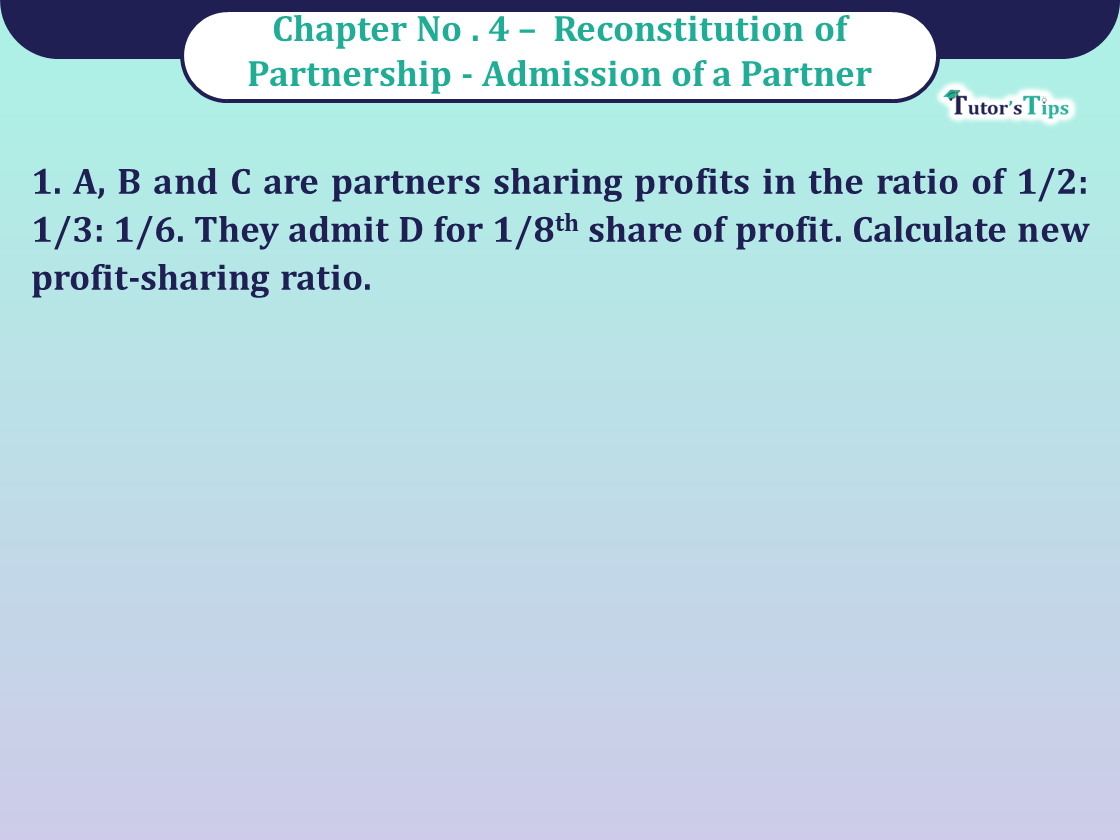# Question 1 Chapter 4 of Class 12 Part – 1 VK PublicationQuestion 1 Chapter 4 of Class 12 Part - 1 VK Publication

Question 1 Chapter 4 of Class 12 Part – 1

1. A, B and C are partners sharing profits in the ratio of 1/2: 1/3: 1/6. They admit D for 1/8th share of profit. Calculate new profit-sharing ratio.

## The solution of Question 1 Chapter 4 of Class 12 Part – 1: –

Old Ratio of A, B and C = 3:2:1
D is admitted for 1/8th share
Let the total profit = 1

 D’s Share = 1 8

 Remaining Profit = 1 – 1 8
 = 7 8 A’s New Share = 3 x 7 6 8
 = 21 48
 B’s New Share = 2 x 7 6 8
 = 14 48

 C’s New Share = 1 x 7 6 8
 = 7 48
 D’s New Share = 1 or 6 48

New Ratio of A, B, C and D = 21:14:7:6

Comment if you have any questions.

Also, Check out the solved question of previous Chapters: –